The data is formed from:

Item_Identifier: Unique product ID Item_Weight: Weight of product Item_Fat_Content: Whether the product is low fat or not Item_Visibility: The % of total display area of all products in a store allocated to the particular product Item_Type: The category to which the product belongs Item_MRP: Maximum Retail Price (list price) of the product Outlet_Identifier: Unique store ID Outlet_Establishment_Year: The year in which store was established Outlet_Size: The size of the store in terms of ground area covered Outlet_Location_Type: The type of city in which the store is located Outlet_Type: Whether the outlet is just a grocery store or some sort of supermarket Item_Outlet_Sales: Sales of the product in the particulat store. This is the outcome variable to be predicted.

In :
import pandas as pd
import numpy as np
import matplotlib.pyplot as plt
import seaborn as sns
from sklearn.preprocessing import LabelEncoder
import sklearn
from sklearn.linear_model import LinearRegression
from sklearn.metrics import mean_squared_error, r2_score
from sklearn.preprocessing import PolynomialFeatures
from sklearn.preprocessing import StandardScaler
from sklearn.decomposition import PCA
from sklearn.model_selection import KFold, cross_val_score
from sklearn.ensemble import RandomForestRegressor

In :
# merge the training data and test data in one data frame in order to operate on both simultaneously

df = pd.concat([train, test] , ignore_index = True, sort = True)

In :
# get to know our data

df.info()

<class 'pandas.core.frame.DataFrame'>
RangeIndex: 14204 entries, 0 to 14203
Data columns (total 12 columns):
Item_Fat_Content             14204 non-null object
Item_Identifier              14204 non-null object
Item_MRP                     14204 non-null float64
Item_Outlet_Sales            8523 non-null float64
Item_Type                    14204 non-null object
Item_Visibility              14204 non-null float64
Item_Weight                  11765 non-null float64
Outlet_Establishment_Year    14204 non-null int64
Outlet_Identifier            14204 non-null object
Outlet_Location_Type         14204 non-null object
Outlet_Size                  10188 non-null object
Outlet_Type                  14204 non-null object
dtypes: float64(4), int64(1), object(7)
memory usage: 1.3+ MB

Out:
Item_Fat_Content Item_Identifier Item_MRP Item_Outlet_Sales Item_Type Item_Visibility Item_Weight Outlet_Establishment_Year Outlet_Identifier Outlet_Location_Type Outlet_Size Outlet_Type
0 Low Fat FDA15 249.8092 3735.1380 Dairy 0.016047 9.30 1999 OUT049 Tier 1 Medium Supermarket Type1
1 Regular DRC01 48.2692 443.4228 Soft Drinks 0.019278 5.92 2009 OUT018 Tier 3 Medium Supermarket Type2
2 Low Fat FDN15 141.6180 2097.2700 Meat 0.016760 17.50 1999 OUT049 Tier 1 Medium Supermarket Type1
3 Regular FDX07 182.0950 732.3800 Fruits and Vegetables 0.000000 19.20 1998 OUT010 Tier 3 NaN Grocery Store
4 Low Fat NCD19 53.8614 994.7052 Household 0.000000 8.93 1987 OUT013 Tier 3 High Supermarket Type1
In :
# how many unique values each of the variable has should be an interesting information

df.apply(lambda x : len(x.unique()))

Out:
Item_Fat_Content                 5
Item_Identifier               1559
Item_MRP                      8052
Item_Outlet_Sales             3494
Item_Type                       16
Item_Visibility              13006
Item_Weight                    416
Outlet_Establishment_Year        9
Outlet_Identifier               10
Outlet_Location_Type             3
Outlet_Size                      4
Outlet_Type                      4
dtype: int64

What's the relationships between individual features as well as between the features and the dependent variable.

In :
corr = df.corr()

#Heatmaps show the strenght of relationships between the data features.
sns.heatmap(corr, annot=True)
plt.xticks(rotation=45)

Out:
(array([0.5, 1.5, 2.5, 3.5, 4.5]), <a list of 5 Text xticklabel objects>)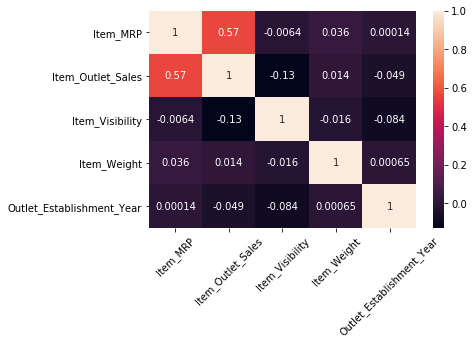We saw previously that we have 2 columns with missing values, let's find the percentage:

In :
percent_missing = df[['Item_Weight', 'Outlet_Size']].isnull().sum() * 100 / len(df)
percent_missing

Out:
Item_Weight    17.171219
Outlet_Size    28.273726
dtype: float64
In :
# I will try to replace the missing values from Item Weight in consideration with Item Type
df["Item_Weight"] = df.groupby("Item_Type")['Item_Weight'].apply(lambda x: x.fillna(x.mean()))

# and those from outlet size considering outlet type
from scipy.stats import mode
df["Outlet_Size"] = df.groupby("Outlet_Type")['Outlet_Size'].apply(lambda x: x.fillna(mode(x.astype('str')).mode)) # when we have categorical why do we need double mode?


Let's draw some plot and see what will find:

In :
plt.hist(df.Item_Weight, bins = 50)
plt.show()
# between 12.50 and 13.50 are the most common weights

#or

plt.hist(df['Item_Visibility'], bins=30)
plt.show()
# are expresed as a percentage? most of them have 3% visibility?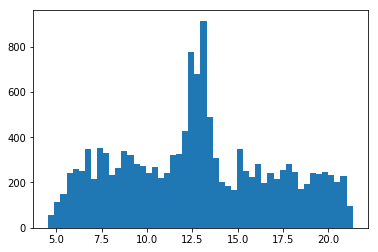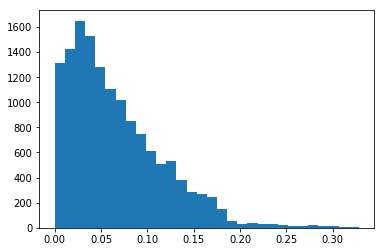One of the most useful plots are the boxplot. The central box of the boxplot represents the middle 50% of the observations, the central bar is the median and the bars at the end of the dotted lines (whiskers) encapsulate the great majority of the observations. Circles that lie beyond the end of the whiskers are data points that may be outliers.

One of the most useful features of a boxplot is the ability to make side-by-side boxplots. A side-by-side boxplot takes a numeric variable and splits it on based on some categorical variable, drawing a different boxplot for each level of the categorical variable.

In :
df.boxplot(column="Item_Outlet_Sales",        # Column to plot
by= "Outlet_Location_Type",         # Column to split upon
figsize= (8,8))        # Figure size

# we can see that tier 3 has a wider range of sales and higher outliers but that's it...

df.boxplot(column="Item_Outlet_Sales",        # Column to plot
by= "Outlet_Size",         # Column to split upon
figsize= (8,8))

# Curious enough there is not that of a high discrepancy between outlet sizes. And even stranger is that the medium
# size outlet

df.boxplot(column="Item_Outlet_Sales",        # Column to plot
by= "Outlet_Type",         # Column to split upon
figsize= (8,8))

# we can see that the sales ar by far higher on the Supermarket Type 3 but what's interesting is that the Type 1 has
# allot of outlier sales

Out:
<matplotlib.axes._subplots.AxesSubplot at 0x1df5fbf9240>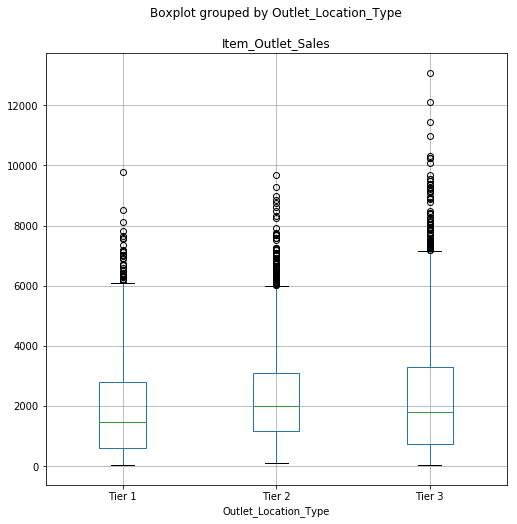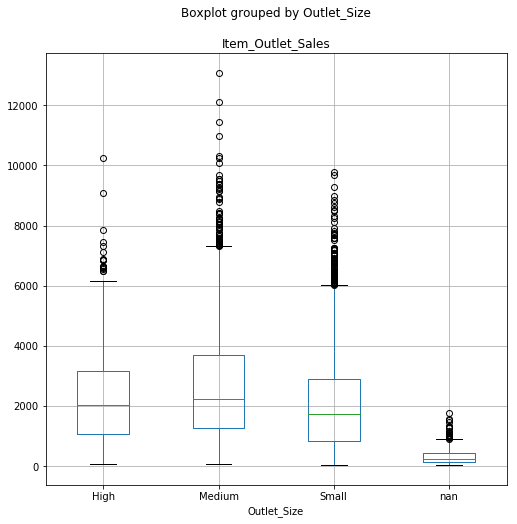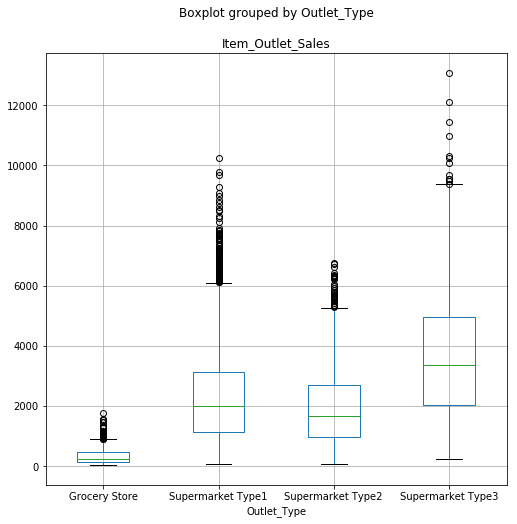In :
df.Item_Fat_Content.value_counts()

Out:
Low Fat    8485
Regular    4824
LF          522
reg         195
low fat     178
Name: Item_Fat_Content, dtype: int64
In :
# we can see that we have 5 categories but with same meaning, let's fix that:

df['Item_Fat_Content'] = df['Item_Fat_Content'].replace({'LF':'Low Fat',
'low fat':'Low Fat',
'reg':'Regular'})
print (df['Item_Fat_Content'].value_counts())

Low Fat    9185
Regular    5019
Name: Item_Fat_Content, dtype: int64


Further more we can see that Item_Visibility has values of 0. How is possible that a product is not visible at all, considering that we are talking about those products that are on the shelf

In :
def replace(x):
if x == 0:
return df.Item_Visibility.mean()
else:
return x

# we can replace those values with the average
df.Item_Visibility = df.Item_Visibility.apply(replace)


If we take a look at the Item_Type there are way too many groups/clusters and that makes it harder for a prediction algorithm. That needs to be changed:

In :
def change(x):
if x in ['Fruits and Vegetables', 'Snack Food', 'Frozen Food', 'Dairy', 'Canned', 'Baking Goods', 'Meat', 'Breads', 'Starchy Foods', 'Breakfast', 'Seafood']:
return 'Food'
elif x == ['Household', 'Health and Hygiene', 'Others']:
return 'Non-Consumable'
else:
return 'Drinks'

df.Item_Type = df.Item_Type.apply(change)

In :
df.Outlet_Establishment_Year.value_counts()

Out:
1985    2439
1987    1553
1999    1550
1997    1550
2004    1550
2002    1548
2009    1546
2007    1543
1998     925
Name: Outlet_Establishment_Year, dtype: int64
In :
# drop item and outlet identifier. They don't help the alghoritm
df = df.drop(['Item_Identifier', 'Outlet_Identifier'], axis = 1)


We got at the prediction part of the problem. This is going to be a supervised machine learning problem. In particular, many machine learning algorithms require that their input is numerical and therefore categorical features must be transformed into numerical features before we can use any of these algorithms. Following the same idea, in order to use the machine learning models we need to create dummy variables for that columns which are categorical.

In :
catg = ['Item_Fat_Content','Outlet_Location_Type','Outlet_Size','Item_Type','Outlet_Type']

In :
le = LabelEncoder()
for i in catg:
df[i] = le.fit_transform(df[i])


One-Hot-Coding refers to creating dummy variables, one for each category of a categorical variable. For example, the Item_Fat_Content has 3 categories – ‘Low Fat’, ‘Regular’ and ‘Non-Edible’. One hot coding will remove this variable and generate 3 new variables. Each will have binary numbers – 0 (if the category is not present) and 1(if category is present). This can be done using ‘get_dummies’ function of Pandas.

In :
df = pd.get_dummies(df, columns=['Item_Fat_Content','Outlet_Location_Type','Outlet_Size','Outlet_Type',
'Item_Type'])

In :
# regroup
train = df[df['Item_Outlet_Sales'].notnull()]
test = df[df['Item_Outlet_Sales'].isnull()]


Feature scaling is a method used to normalize the range of independent variables or features of data:

In :
test = test.drop('Item_Outlet_Sales', axis = 1)

y = train.Item_Outlet_Sales
train = train.drop('Item_Outlet_Sales', axis = 1)
train_list = list(train.columns)

slc= StandardScaler()
train_sc = slc.fit_transform(train)
test_sc = slc.fit_transform(test)

C:\Users\s7c\Anaconda3\lib\site-packages\sklearn\preprocessing\data.py:645: DataConversionWarning: Data with input dtype uint8, int64, float64 were all converted to float64 by StandardScaler.
return self.partial_fit(X, y)
C:\Users\s7c\Anaconda3\lib\site-packages\sklearn\base.py:464: DataConversionWarning: Data with input dtype uint8, int64, float64 were all converted to float64 by StandardScaler.
return self.fit(X, **fit_params).transform(X)
C:\Users\s7c\Anaconda3\lib\site-packages\sklearn\preprocessing\data.py:645: DataConversionWarning: Data with input dtype uint8, int64, float64 were all converted to float64 by StandardScaler.
return self.partial_fit(X, y)
C:\Users\s7c\Anaconda3\lib\site-packages\sklearn\base.py:464: DataConversionWarning: Data with input dtype uint8, int64, float64 were all converted to float64 by StandardScaler.
return self.fit(X, **fit_params).transform(X)


Principal Component Analysis PCA is a tool which helps to produce better visualizations of high dimensional data. So, we need to calculate the variance of each variable we are given. Then drop the variables having low variance as compared to other variables in our dataset. The reason for doing this, as I mentioned above, is that variables with a low variance will not affect the target variable. First principal component is a linear combination of original predictor variables which captures the maximum variance in the data set. It determines the direction of highest variability in the data. Larger the variability captured in first component, larger the information captured by component. No other component can have variability higher than first principal component. The first principal component results in a line which is closest to the data i.e. it minimizes the sum of squared distance between a data point and the line.

In :
pca = PCA(n_components=None)
train = pca.fit_transform(train_sc)
test = pca.fit_transform(test_sc)

#Plotting the Cumulative Summation of the Explained Variance
plt.figure()
plt.plot(np.cumsum(pca.explained_variance_ratio_))
plt.xlabel('Number of Components')
plt.ylabel('Variance (%)') #for each component
plt.title('Dataset Explained Variance')
plt.show()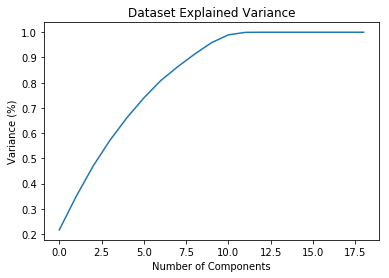In :
# this graph tells us that with 10 components we preserve around 90% of the data

pca = PCA(n_components = 10)
train = pca.fit_transform(train_sc)
test = pca.fit_transform(test_sc)


First we need to train our model in order to learn the relationships between the features and the target The next step is figuring out how good the model is! To do this we make predictions on the test features (the model is never allowed to see the test answers). We then normally compare the predictions to the known answers. When performing regression, we need to make sure to use the absolute error because we expect some of our answers to be low and some to be high. We are interested in how far away our average prediction is from the actual value so we take the absolute value.

Multi-linear regression Model. It's a linear regression with multiple variables to predict.

In :
ln = LinearRegression()
ln.fit(train,y)
# predict
print ('Coefficients: ', ln.coef_)
# Coefficient and Intercept , are the parameters of the fit line

y_predicted = ln.predict(test)

Coefficients:  [  23.02530996  -21.04452783 -377.88764689 -305.26273469  -44.71939823
8.33333469  291.08329168  690.43798941  688.42366499   97.73838323]


Polynomial regression PolynomialFeatures() function in Scikit-learn library, drives a new feature sets from the original feature set. Polynomial regression is considered to be a special case of traditional multiple linear regression. So, you can use the same mechanism as linear regression to solve such a problems.

In :
from sklearn.preprocessing import PolynomialFeatures
from sklearn.model_selection import KFold, cross_val_score
poly = PolynomialFeatures(degree=4) #These 3 steps are to convert X matrix into X polynomial
train_poly=poly.fit_transform(train) #matrix.
regressor_poly = LinearRegression()
regressor_poly.fit(train_poly,y)

Out:
LinearRegression(copy_X=True, fit_intercept=True, n_jobs=None,
normalize=False)
In :
# Let us check the accuracy
accuracy = cross_val_score(estimator=regressor_poly, X=train, y=y,cv=10)
print(f"The accuracy of the Polynomial Regression Model is \t {accuracy.mean()}")
print(f"The deviation in the accuracy is \t {accuracy.std()}")

The accuracy of the Polynomial Regression Model is 	 0.5413630439593914
The deviation in the accuracy is 	 0.020700864427139535


Random Forest A random forest is a data construct applied to machine learning that develops large numbers of random decision trees analyzing sets of variables. This type of algorithm helps to enhance the ways that technologies analyze complex data.

In :
# Import the model
from sklearn.ensemble import RandomForestRegressor
# Instantiate model with 100 decision trees
rf = RandomForestRegressor(n_estimators = 100, random_state = 21)
# Train the model on training data
rf.fit(train, y);

predictions = rf.predict(test)

# Get numerical feature importances
importances = list(rf.feature_importances_)
# List of tuples with variable and importance
feature_importances = [(feature, round(importance, 2)) for feature, importance in zip(train_list, importances)]
# Sort the feature importances by most important first
feature_importances = sorted(feature_importances, key = lambda x: x, reverse = True)
# Print out the feature and importances
[print('Variable: {:20} Importance: {}'.format(*pair)) for pair in feature_importances];

Variable: Outlet_Location_Type_2 Importance: 0.26
Variable: Outlet_Location_Type_1 Importance: 0.24
Variable: Item_Weight          Importance: 0.2
Variable: Item_MRP             Importance: 0.06
Variable: Outlet_Location_Type_0 Importance: 0.06
Variable: Item_Visibility      Importance: 0.04
Variable: Item_Fat_Content_0   Importance: 0.04
Variable: Item_Fat_Content_1   Importance: 0.04
Variable: Outlet_Size_0        Importance: 0.04
Variable: Outlet_Establishment_Year Importance: 0.03